• 相机标定的目的和意义
• 相机成像过程的简化与建模
• 针孔相机模型的数学描述
• 标定针孔相机模型的参数

## 相机标定的目的和意义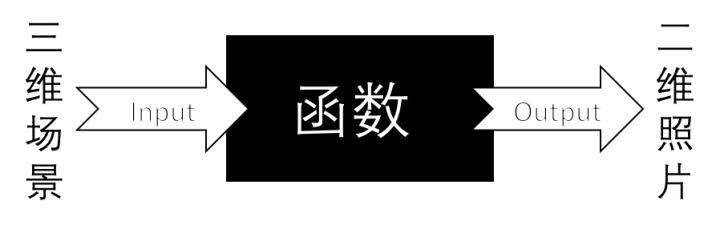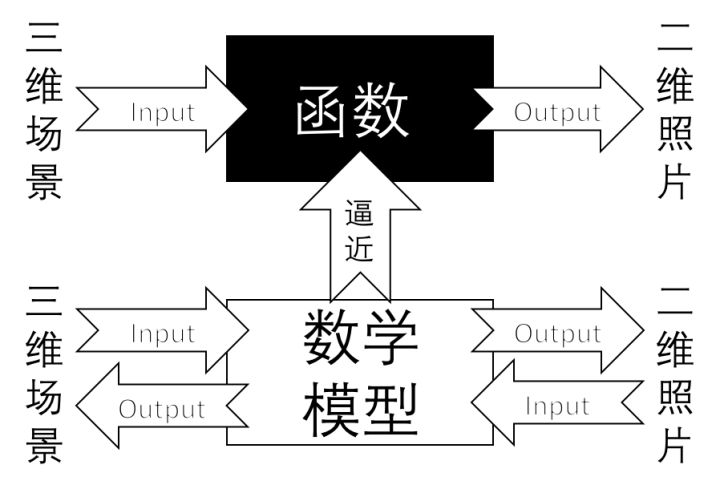## 相机成像过程的简化与建模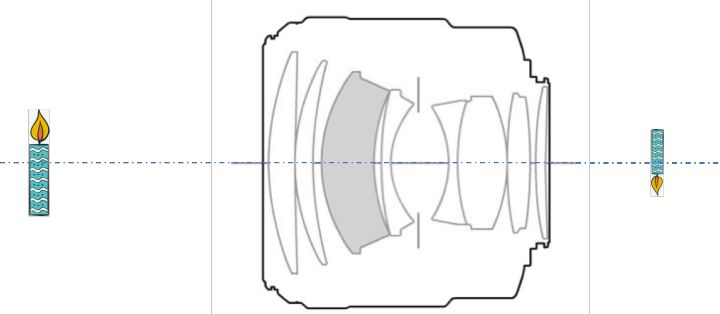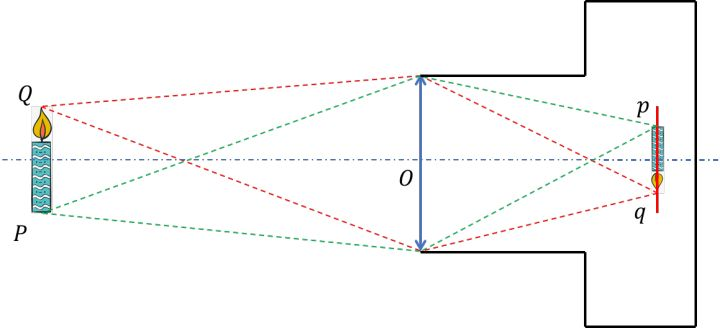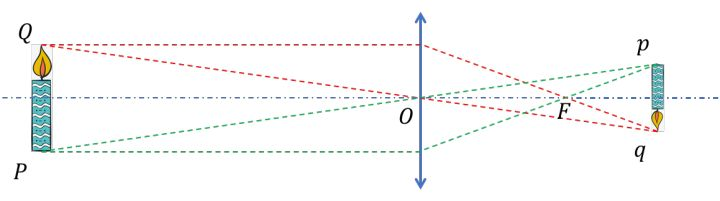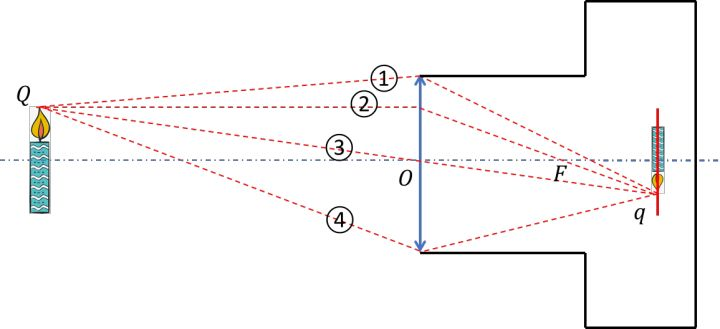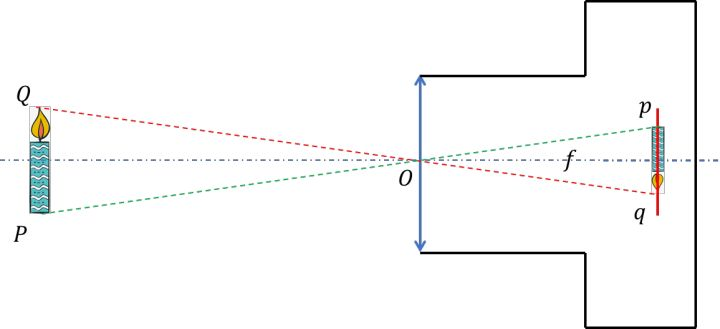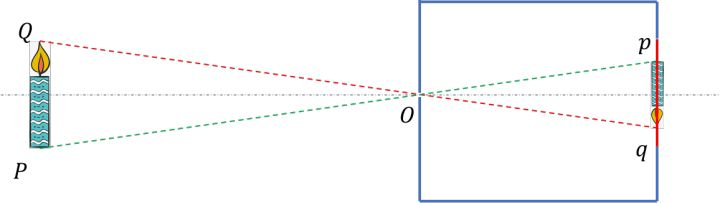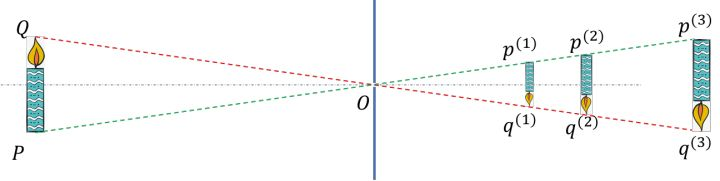## 针孔相机模型的描述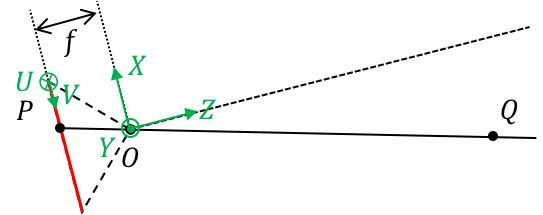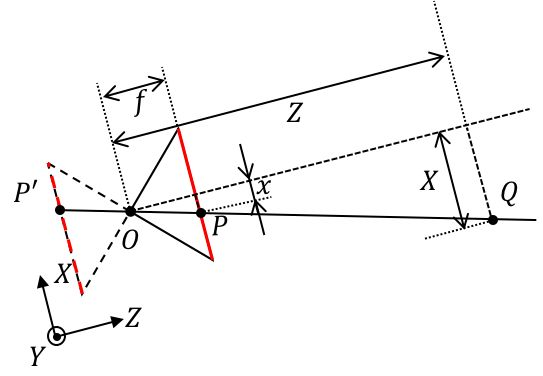• 由光心 O 沿着光轴出发，像平面在 Z=f 上， f 是相机的物理焦距（单位： mm）。
• 点 Q 在空间中，在相机坐标系下的位置是$Q(X,Y,Z)$。
• 点 P 在像平面上，有两个等价的位置描述：1. 在相机坐标系下的位置是 P(x,y,f) ；2. 在CCD标号坐标系下的位置是 $P(u_{ccd},v_{ccd})$。
• 在无镜头畸变的条件下，光心 O 、点 P 与点 Q 在一条直线上。
• k 与 l 是CCD单个像素在水平和竖直两个方向上的尺寸（单位： mm /像素），因此定义焦距为 $$f_x=\frac{f}{k},f_y=\frac{f}{l}$$ （单位：像素）。
• CCD标号坐标系原点到光轴的偏移量为 $c_x,c_y$ （单位：像素）。

(1) 二维CCD标号坐标(u_{ccd},v_{ccd})$$\leftrightarrow二维CCD像素坐标 (x,y[,f]) 建立CCD像面上的点标号坐标与物理坐标之间关联，由于像平面在 Z=f上，可省略。$$\left(\begin{array}{c}u_{ccd}\v_{ccd}\1\end{array}\right)=\left(\begin{array}{c}\frac{1}{k}&0&c_x\0&\frac{1}{l}&c_y\0&0&1\end{array}\right)\left(\begin{array}{c}x\y\1\end{array}\right)$$(2) 像点的二维像素坐标 (x,y)\leftrightarrow 物点的三维空间中的坐标 (X,Y,Z) 建立CCD像面上像点物理坐标与对应的三维空间中物点坐标之间关联。$$\left(\begin{array}{c}xZ\yZ\Z\end{array}\right)=\left(\begin{array}{c}f_xk&0&0\0&f_yl&0\0&0&1\end{array}\right)\left(\begin{array}{c}X\Y\Z\end{array}\right)$$(3) 像点的二维CCD标号坐标 (u_{ccd}, v_{ccd})\longleftrightarrow 物点的三维空间中的坐标 (X, Y, Z) 连接(1)和(2)的两个关联，也是实际进行标定得到参数 f_x, f_y, c_x, c_y$$\left(\begin{array}{c}u_{ccd}*Z\v_{ccd}*Z\Z\end{array}\right)=\left(\begin{array}{c}f_x&0&c_x\0&f_y&c_y\0&0&1\end{array}\right)\left(\begin{array}{c}X\Y\Z\end{array}\right)$$由以上三个关联可以得到：像点标号 (u_{ccd},v_{ccd})\longleftrightarrow像点坐标 (x, y, f)\longleftrightarrow 物点坐标 (X, Y, Z)。 在OpenCV及Matlab标定工具箱直接使用了(3)关联，不需要知道CCD单个像素的尺寸，因此在标定过程是得不到物理焦距 f 的，只能得到像素焦距 f_x,f_y。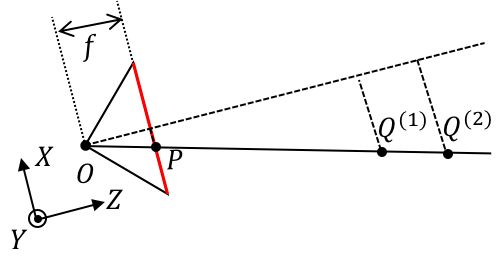很容易发现，(3)关联是不约束 Z 的，也就是说，针孔相机模型本身是欠定的，通过被点亮的CCD像素 P 我们只能知道物点 Q^{(?)} 在射线 \overline{OP} 上，但无法确定具体的点，所以我们讲，针孔相机模型是一个射线方程模型，\overline{OP} 点的射线方程：$$\left(\begin{array}{c}x\y\z\end{array}\right)=\lambda\left(\begin{array}{c}x_P\y_P\f\end{array}\right)$$以上的关系是在无镜头畸变的条件下建立的，但是实际上存在镜头畸变，可以理解成像点和物点之间的光线是弯曲的，要得到射线模型，要进行消除畸变。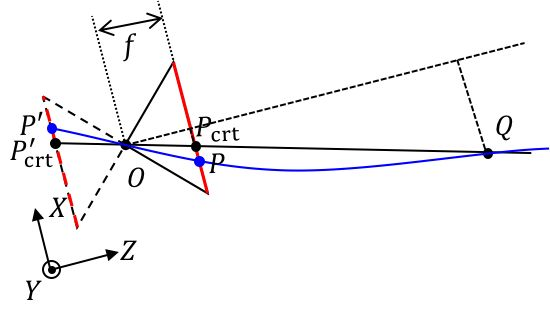(4) 补充消畸变模型 以像面中心像素坐标 (0,0,f) 为中心，像面上的点到中心的距离为 r=\sqrt{x^2+y^2} • 合成畸变$$\left{\begin{aligned}x_{crt}=x_{rcrt}+x_{tcrt}\y_{crt}=y_{rcrt}+y_{tcrt}\end{aligned}\right.$$其中： • 径向畸变$$\left{\begin{aligned}x_{rcrt}=x(1+k_1r^2+k_2r^4+k_3r^6)\y_{rcrt}=y(1+k_1r^2+k_2r^4+k_3r^6)\end{aligned}\right.$$• 切向畸变$$\left{\begin{aligned}x_{tcrt}=x+[2p_1y+p_2(r^2+2x^2)]\y_{tcrt}=y+[p_1(r^2+2y^2)+2p_2x]\end{aligned}\right.$$把消畸变模型补充进针孔相机模型，因此「像点标号 (u_{ccd},v_{ccd})\longleftrightarrow 像点坐标物点坐标 (X, Y, Z) 被修正为： 像点标号 (u_{ccd-crt},v_{ccd-crt})\longleftrightarrow像点坐标 (x_{crt}, y_{crt}, f)\longleftrightarrow物点坐标 (X,Y,Z) 消畸变后的射线方程 \overline{OP_{crt}}是：$$\left(\begin{array}{c}x\y\z\end{array}\right)=\lambda\left(\begin{array}{c}x_{P_{crt}}\y_{P_{crt}}\f\end{array}\right)$$## 标定针孔相机模型的参数 针孔相机模型中，只要确定这9个参数就可以唯一的确定针孔相机模型f_x,f_y,c_x,c_y,k_1,k_2,k_3,p_1,p_2​ ，这个过程就称为「相机标定」，其中前4个我们称为内参数，后5个称为畸变参数，畸变参数是为了补充内参的。所以一旦相机结构固定，包括镜头结构固定，对焦距离固定，我们就可以用这9个的参数去近似这个相机。这里说的「镜头结构固定」，按我个人的理解，除了焦距固定之外，也应当包含光圈固定，因为改变光圈的大小，除了景深之外，是有可能改变针孔相机模型中的光心位置，但是影响并不是很大。这意味着标定好的相机如果改变光圈大小，会使得标定误差变大但应该不会大到难以接受的地步。 对于针孔相机本身需要拟合的方程如下：$$\left(\begin{array}{c}u_{ccd_{crt}}*Z\v_{ccd_{crt}}*Z\Z\end{array}\right)=J(k_1,k_2,k_3,p_1,p_2)\left(\begin{array}{c}f_x&0&c_x\0&f_y&c_y\0&0&1\end{array}\right)\left(\begin{array}{c}X\Y\Z\end{array}\right)$$其中 J(k_1,k_2,k_3,p_1,p_2)代表了畸变图像和消畸变图像之间的变换。 因此，我们现在的任务就是找出一大堆具有对应关系的像点 {(u_{ccd_{crt}},v_{ccd_{crt}})^T} 和物点 {(X,Y,Z)^T}的点对作为样本，来训练出模型的9个参数f_x,f_y,c_x,c_y,k_1,k_2,k_3,p_1,p_2 。那么这里就引发了两个问题：(1) 这么多像点和物点到底谁和谁是一对儿？(2) 即便我知道物点在哪里，可是我怎么样用相机坐标系来表达物点的位置 (X,Y,Z)? 为了解决上述的两个问题，标定板应运而生。标定板的第一大作用，确定物点和像点的对应性。这里用到的原理主要是「透视不变性」，打个比方，你近看一个人和远看一个人，虽然他的鼻子大小变了，你看鼻子的视角也变了，但是拓扑结构肯定是不变的，你也不可能把鼻子看成是嘴巴。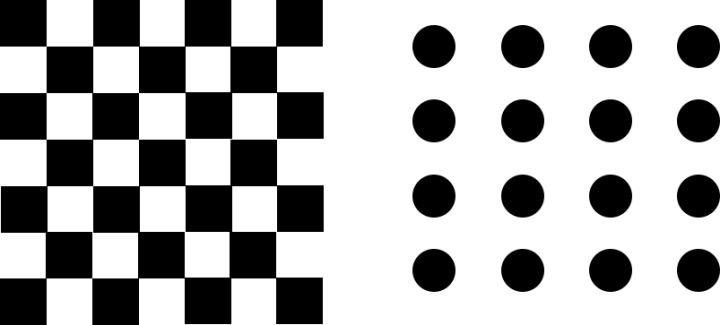所以在标定板中，印刷了拓扑结构，广泛应用的是棋盘格和圆点格，这两种之所以成为主流，不仅是因为它们的拓扑结构明确且均匀，更重要的是检测其拓扑结构的算法简单且有效。棋盘格检测的是角点，只要对拍摄到的棋盘格图像横纵两个方向计算梯度就可获得；而圆点格的检测只需要对拍摄到的圆点格图样计算质心即可。假如你开发了一套非常完美的检测人脸全部特征的算法，你完全可以用你的照片当作标定板。 按照我的经验，圆点格的效果应该是好于棋盘格，因为圆点质心的「透视不变性」要比棋盘格的角点稳定的多。下图是同样尺寸、同样比例棋盘格和圆点在最大重投影误差处的误差对比，红色十字是提取的角点/质心，绿色圆圈是针孔相机模型计算出来认为的角点/质心位置。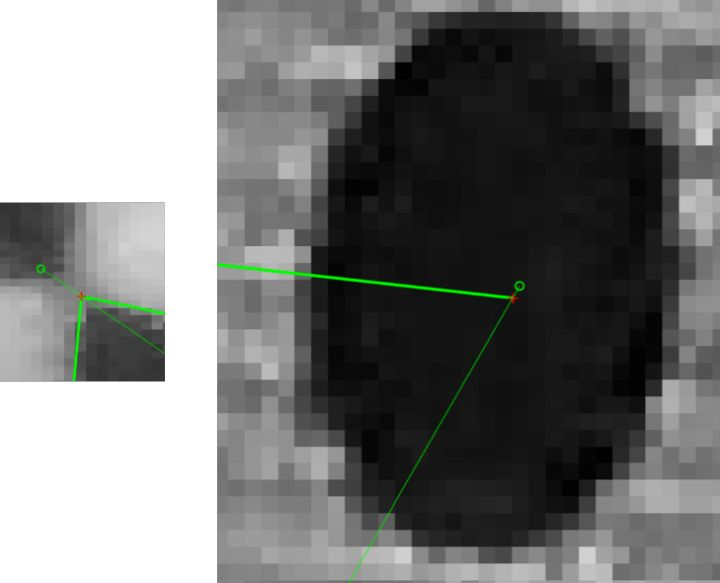下图是棋盘格与圆点格的重投影误差图，显然圆点格的重投影误差的误差空间要小。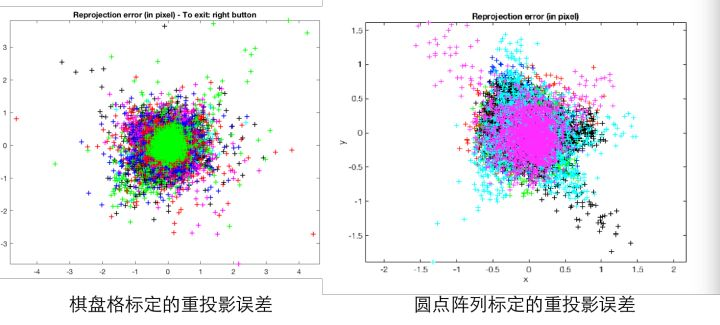但是圆点格的检测似乎是Halcon的专利（存疑），因此OpenCV和Matlab标定工具箱用的是棋盘格，要用圆点格得要自己写算法。下文中提到的标定板说的都是棋盘格。 标定板的第二大作用是把标定板中的角点变换到相机坐标系下的坐标 (X,Y,Z)。对于标定的初学者来说，很容易忽略的一点是标定板是具有标定板坐标系的。换句话说，标定板中的每个角点，在标定板坐标系下的位置是确定并且是已知的。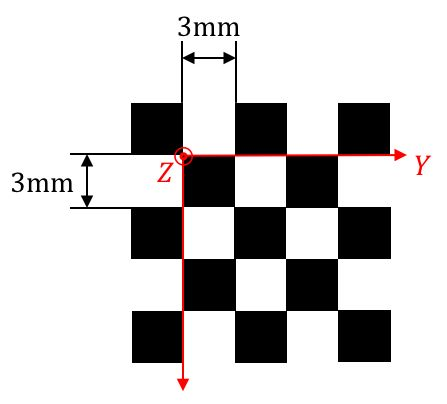而标定板坐标系变换到相机坐标系的变换矩阵，我们称它的元素为外参数，所以在我眼中，相机标定的外参根本就是标定内参的副产品，它会随着标定板的摆放不同而不同，由标定板坐标系变换到相机坐标系的变换矩阵可以由下式表达：$$\left(\begin{array}{c}X_C\Y_C\Z_C\end{array}\right)=R_{b2C}\left(\begin{array}{c}X_b\Y_b\Z_b\end{array}\right)+T_{b2C}$$其中， R_{b2C} 称为旋转矩阵，T_{b2C}称为平移矩阵，下表b2C代表board to camera。注意这个坐标系的变换是一个线形变换，反应到物理上讲，这意味者标定板必须尽可能的平，如果标定板不平，这个变换就不是线形的了。我们把这个变换带到原本要拟合的方程中去：$$\left(\begin{array}{c}u_{ccd_{crt}}\v_{ccd_{crt}}\end{array}\right)=J(k_1,k_2,k_3,p_1,p_2)\left(\begin{array}{c}f_x&0&c_x\0&f_y&c_y\0&0&1\end{array}\right)\left(R_{b2C}\left(\begin{array}{c}X_b\Y_b\Z_b\end{array}\right)+T_{b2C}\right)$如此一来，我们就把能够把CCD上拍摄的到角点的像素标号$(u_{ccd_{crt}},v_{ccd_{crt}})$和每一个角点已知的在标定板坐标系下的坐标$(X_b,Y_b,Z_b) $对应起来，通过各种各样的姿态作为样本，训练出全部参数$f_x,f_y,c_x,c_y,k_1,k_2,k_3,p_1,p_2,R_{b2C},T_{b2C}\$ 。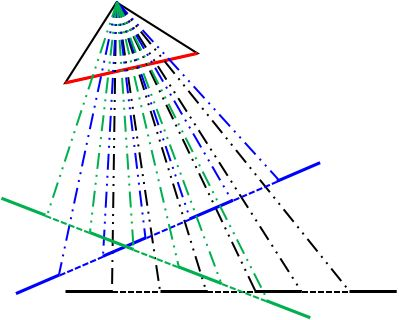1. 标定板的平面度高，棋盘格是直角；
2. 标定板每个格子尺寸的高一致性；
3. 真实尺寸与标称尺寸的差异小。

A Flexible New Technique for Camera Calibration

【机器视觉】张氏法相机标定